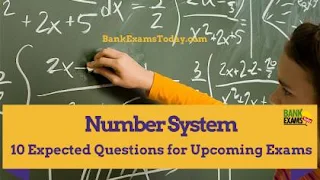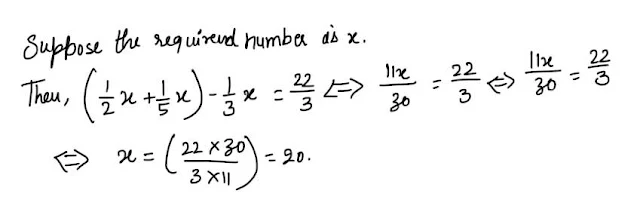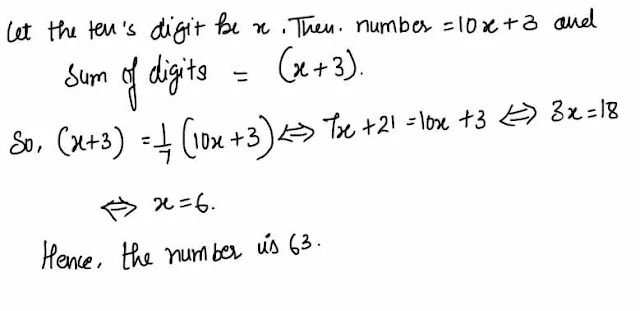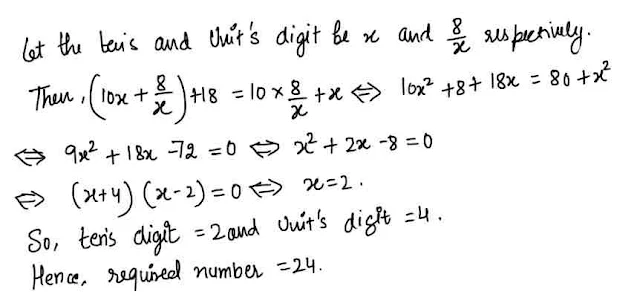New Student User - Use Code HELLO

# Number System : 10 Expected Questions for Upcoming Exams#### Ques 1.

The difference between a two- digit number and the number obtained by interchanging the two digit is 63. Which is the smaller of the two numbers ?

(a)29
(b) 70
(c)92
(d) Cannot be determined
Ans 1. Let the ten's digit be x and unit's be y .
Then. ( 10x + y) - ( 10x + x) = 63 ⇔ 9 ( x- y) = 63 ⇔ x - y = 7
Thus, none of the numbers can be determined.

#### Ques 2.

A certain number of two-digit is three times the sum of its digit and if 45 be added to it, the digit are reversed .The number is :
(a) 23
(b) 27
(c) 32
(d) 72

Ans 2. Let the ten's digit be x and unit's digit be y.
Then, 10x + y = 3 ( x+ y)   ⇔ 7x - 2y = 0
10x + y + 45 = 10y + x ⇔ y -x = 5
Solving (i) and ( ii), we get : x = 2 and y = 7.
Required number = 27.

#### Ques 3.

The sum of three numbers is 264.If the first number be twice the second and third number be one-third of the first ,then the second number is :
(a) 48
(b) 54
(c) 72
(d) 84
Ans 3.#### Ques 4.

The Product of two number is 192 and the sum of these of these two numbers is 28. What is the smaller of these two numbers ?
(a) 12
(b) 14
(c) 16
(d) 18
Ans 4.   12

#### Ques 5.

The difference between two positive integers is 3.If the sum of their squares is 369, then the sum of the numbers is :
(a) 25
(b) 27
(c) 33
(d) 81
Ans 5.Suppose the required number is x. Then from question,
x2 + ( x + 3)2  = 369 ⇔ x2 + x2 + 9 + 6x = 369
⇔ 2x2 + 6x - 360 = 0 ⇔  x2 + 3x - 180 = 0
⇔ ( x+15) (x -12) = 0  ⇔ x = 12
So, The numbers are 12 and 15.
∴ Required sum = ( 12 + 15) = 27.

#### Ques 6.

The sum of the digit of a two-digit number is 15 and the difference between the digit is 3. What is the two- digit number ?
(a) 69
(b) 78
(c) 96
(d) Cannot be determined
Ans 6. Cannot be determined

#### Ques 7.

Find a positive number which when increased by 17 is equal to 60 times the reciprocal of the number.
(a) 3
(b) 10
(c) 17
(d) 20
Ans 7.  3

#### Ques 8.

One -fifth of a number is equal to 5/8 of another number. If 35 added to the first number,it becomes four times of the second number. The second number is :
(a) 25
(b) 40
(c) 70
(d) 125
Ans 8.#### Ques 9.

If the sum of one-half and one-fifth of a number exceeds one-third of that number by 7 1/3, the number is :
(a) 15
(b) 18
(c) 20
(d) 30
Ans 9. Suppose the required number is x.#### Ques 10.

A number of two digit has 3 for its unit's digit,and the sum of digit is 1/7 of the number itself. The number is
(a) 43
(b) 53
(c) 63
(d) 73
Ans 10.#### Ques 11.

The sum of three consecutive odd numbers is 20 more than the first of these numbers. What is the middle number ?
(a) 7
(b) 9
(c) 11
Ans 11. Suppose the three numbers are x, x + 2  and x + 4
The, x + ( x+ 2) + ( x+ 4) = x + 20 ⇔ 2x = 14 ⇔ x = 7.
∴ Middle number = x + 2 = 9.

#### Ques 12.

A two-digit number is such that the product of the digit is 8. When 18 is added to the number , then the digit are reversed. The number is :
(a) 18
(b) 24
(c) 42
(d) 81
Ans 12.#### Ques 13.

The sum of the squares of three numbers is 138, while the sum of their products taken two at a time is 131.Their sum is :
(a) 20
(b) 30
(c) 40
(d) None of these
Ans 13.